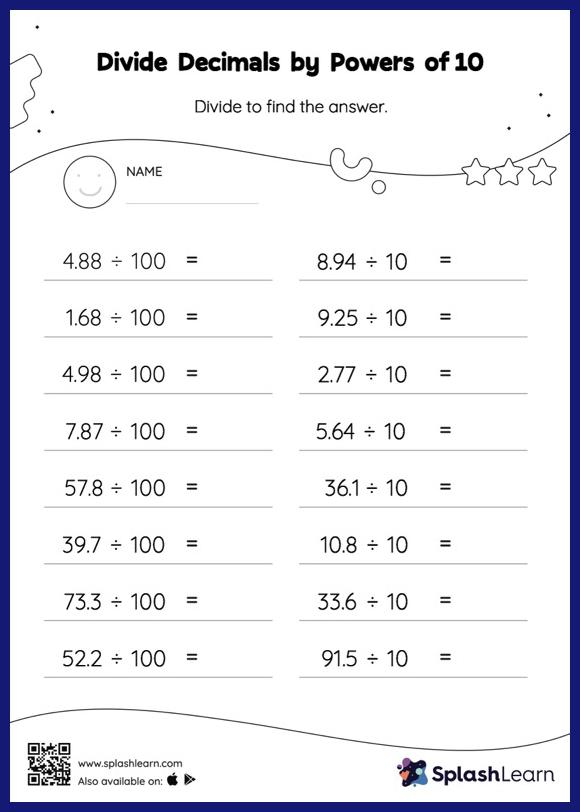# Divide Decimals by Powers of 10: Horizontal Division Worksheet

Home > Divide Decimals by Powers of 10: Horizontal DivisionGive your little one some practice building division skills with this divide decimals by powers of 10 worksheet. When a decimal is divided by a power of 10, the decimal point moves to the left by the same amount as the number of zeros in the power of 10. Students practice this concept in great depth with divide decimals by powers of 10 worksheet. In this worksheet, students practice solving problems written in the horizontal format. How numbers are laid out in a problem affects the method a student employs to solve it. Therefore to develop actual fluency and mastery of multiple strategies, students must practice different formats.